• Submissions# A Simplified Approach for Predicting the Time of Consolidation for a Multi-Layered Foundation

Tham H Duong*

Department of Civil Engineering, Ho Chi Minh City Open University, Vietnam

*Corresponding author: Tham H Duong, Department of Civil Engineering, Ho Chi Minh City Open University, Vietnam

Submission: September 21, 2021;Published: September 28, 2021

DOI: 10.31031/RDMS.2021.15.000871ISSN: 2576-8840
Volume 15 Issue 5

#### Abstract

This paper deals with a simplified approach of predicting the time of consolidation settlement for a multi-layered foundation using the coupling technique for settlement and unsettlement zones in a specific compressible layer and layers. According to MIT’s lecture, every two layers should combine into one having an equivalent degree of consolidation or having an equivalent thickness of consolidation. From this valuable guideline, several procedures are developed to tackle the specific problem of the settlement of a multi-layered foundation. This study also introduces an idea for coupling the layers, concerning the drainage path during combining every two layers, and some practical points are suggested.

Keywords: Consolidation settlement; Equivalent coefficient of consolidation Cv,eq; Thickness Heq; Settlement zones

#### Introduction

Estimating the consolidation settlement of a layered foundation is a complicated task. In multi-layered foundation, and the rate of consolidation settlement for such a multi-layered foundation is a non-linear problem approximately solved by many research works such as: Taking the varied compressibility into account ; considering the variation in permeability . A technique of coupling every two compressive layers into one equivalent is developed by applying MIT’s suggestion .

During the time of consolidation, there are the settlement zones 1a, 2a, and unsettled zones 1b, 2b or still zones recognized (Figure 1), and the compressibility of the zone of settlement (i.e., 1a for layer 1 and 2a for layer 2) has definitely varied, both in permeability and compressibility. By assuming that the unsettled layer (i.e., 1b or 2b in Figure 1) has no variation in the compressibility so that each compressible layer has two separate sub-layers (e.g., 1a and 2a of layer 1), which the coefficient of consolidation is not the same as the original values. A question is what occurs if the zone of settlement, e.g., 1a or 2a, which both have changed the compressibility and the coefficient of consolidation could take into account.

This paper aims to further some results studied by [3,4] investigate the consolidation settlement of the multi-layered compressible foundation, concerning the settlement zones into account.

Figure 1: Settlement zones in a compressible layer.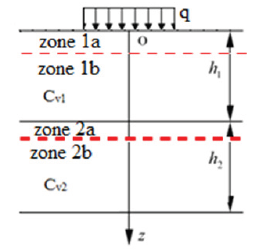#### Theoretical Background

Assumptions

a) Every multi-layered foundation has compressible layers and very low compressible layers (e.g., sand, even a thin layer). The sand layer plays the role of a drainage boundary. As a default concept, the other boundary of drainage is the ground surface.

In the other words, in most possible cases, there is a pervious layer in the foundation

b) The coefficient of consolidation Cv is time-variant quantity, for a varied coefficient of permeability k as expressed in the following equation 1:c) The coefficient of volume compressibility mv also varies with respect to void ratio, therefore a time-variant variable.

d) Coefficient of consolidation Cv will change its value within a settlement zone while being constant if outside the settlement zone (zone 1b in Figure 1).

e) Each layer has its constant characteristics of compressibility and permeability. The load is fully constructed at the start of loading, and soil is normally consolidated. The condition of equivalence is the two models have the same degree of consolidation.

f) The permeability varies only within the settled zone (i.e., zone 1a or 2a); the coefficient of permeability k would be of 3-order power of the void ratio, i.e. k α e3;

g) The Terzaghi’s theory of 1-D consolidation is still valid within a compression layer.

Equivalent coefficient of consolidation

The double-layered foundation are converted to a single layer having the equivalent coefficient of consolidation from the equation (2) without changing the thickness of the foundation (Figures 2 & 3).

Figure 2: Concept of equivalent coefficient of consolidation Cv, eq.Figure 3: The equivalent depth suggested by MIT (Source: MIT’s Lecture Notes).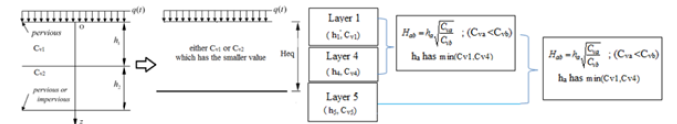Equivalent thickness of consolidation settlement

MIT suggested that an alternative keeps the properties as original values but changes the depth into an equivalent one having the same compressibility (the smaller value of Cv is taken into account) .

The key assumption for the latter approach is that both the boundaries are either pervious or impervious, so that the drainage length would be d = Heq/2 in which Heq is the equivalent thickness of the converted two-layer foundation.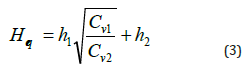where h1 are the thickness having the smaller value of the coefficient of consolidation, or Cv1. Heq then is the equivalent thickness of the foundation that has the greater value of the coefficient of consolidation Cv2.

A simplified model for estimating the degree of consolidation

Fine-grain soil as clay would be assumed to be timedependently compressible, while non-cohesive soil like sand will not be compressible. The multi-layered foundation with stratums are described in Figure 4:

In each layer, the settlement, denoted s1 or s2, is easily predictable as the accumulated vertical deformation over the total thickness of the layer, and then there is an unsettlement zone beneath the settlement zone in this layer, (H1-s1) or (H2-s2) as in Figure 4. Cv,eq1 is the equivalent coefficient of consolidation combining the compressibility of sub-layer s1, and the unsettlement zone (H1-s1) having original Cv,1; Cv,i-j is of the combined lower compressible layers. These equivalent converted layers with sublayers take into account the boundaries of drainage. There are two main patterns of combination: lower and upper equivalent layers which are open layers in the upper part and half-open in the lower part of the foundation. Pattern 1 has the coupled lower conversion, whilst pattern 2 has the upper conversion .

Figure 4: Two possible cases for the consolidation settlement, estimated by the formulas 2.The time of consolidation for the two possible cases is mainly different due to the drainage condition, i.e. the length of drainage d and the effective normal stress in layers. This concern points out that formula (2) using the coefficient of consolidation Cv in prediction is more practicable and reasonable than formula (3) with Heq.

The degree of consolidation, U (%) could be estimated by formula 4a and 4b, as below:where Tv is the dimensionless time factor, determined by the formula, in which d is the drainage length in each layer, and t is the time of consolidation.

A model for validation

The equivalent coefficient Cv,eq and the equivalent thickness Heq are calculated by the formula (2) and (3). The following model will apply it in calculating the consolidation, concerning the compressibility of settlement zones.

Real soft soil properties at a real site in District 2 Ho Chi Minh City (a typically soft soil foundation) are reviewed as in Table 1. As per the Terzaghi’s assumption for the case in which the loading width extends to infinity or very large as compared to the thickness of compressible layer, the 1D consolidation is applicable. The site subjected a 1D consolidation with total stress 32 kPa over a very wide area (nearly 2 meters backfill height over very soft soil foundation, spreading over a very wide area of backfilling) (Figures 5 & 6).

Data are input into the Plaxis 2D model using Mohr Coulomb model, with drained mode for consolidation analysis.

Table 1: Soil layers in this study.Figure 5: Plaxis model.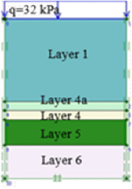Figure 6: Input data for 1D Consolidation Analysis using Plaxis 2D for comparison purpose.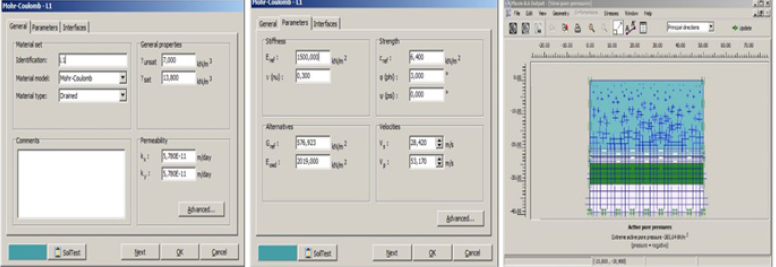Consolidation settlement

Under the overburden pressure po (e.g layer 1, po=43.4kPa) and additional pressure Δp =32kPa, with properties and soil stiffness, the total settlement calculated and described in Table 2.

Table 2: Soil properties and total consolidation settlement.Equivalent coefficient Cv,eq and thickness Heq

Case 1: Taking settlement zone 1a, 2a (Figure 1) into account, calculating the equivalent coefficient of consolidation Cv,eq.

Case 2: In each layer, the settlement zone and the rest part (unsettlement zone) will be converted to a new layer and so on.

#### Comparative Results of Consolidation Settlement

According to the results of the abovementioned convert in Table 3, the time-dependent settlement could be computed by Terzaghi’s theory of 1D consolidation, results are described in Table 4. A finite element model is created with Plaxis 2D V.8.5 as in Figure 2, with Mohr-Coulomb model of analysis for comparison purpose. The Plaxis output of the vertical displacement is shown in Figure 4a and the time-dependent settlement of consolidation is plotted as in Figure 4b.

Table 3: Equivalent values of coefficient of consolidation Cv,eq and thickness Heq.Table 4: Consolidation settlement up to 500 days (settlement in cm).#### Discussion

a) Results obtained by numerical model Plaxis 2D used the update meshing for large strain indicate a nearly perfect 1-dimension consolidation (c.f Figure 7a & 7b).

b) It is tentatively recognized that coupling the layers concerning the settlement zones might result in a greater value of consolidation settlement; the converted foundation which uses the equivalent Cv, eq agrees with the results given by the numerical model, i.e Plaxis©, and predicts a longer time of consolidation. As such it is on the safer side for warning purposes.

c) The degree of consolidation might be different between the two approaches of equivalent foundation, especially when the soil layers are highly compressible, the soft soil layers are thick and the technique of equivalent coefficient of consolidation should be studied with care.

Figure 7: 1D consolidation with vertical deformation and results of time-dependent settlement with/without concerning settlement zones.#### Conclusion

Consolidation settlement could be approximately estimated by using an equivalent coefficient of consolidation or equivalent thickness of compressible layers according to MIT’s approximation. This study suggests every two layers will be coupled to convert them into one single and the settled zone may be viewed as a layer having varied compressibility. An equivalent foundation should use the equivalent coefficient of consolidation Cv,eq instead of the equivalent thickness Heq for a safer prediction. This idea of this study which takes the settlement zone into account and the equivalent coefficient of consolidation should be studied further for predicting the time-dependent settlement in the long term.

1. Miao L, Wang X, Kavazanjian EJ (2008) Consolidation of a double-layered compressible foundation partially penetrated by deep mixed columns. Journal of Geotechnical and Geoenvironmental Engineering 134(8): 1210-1214.
2. Abbasi N, Rahimi H, Javadi AA, Fakher A (2006) Finite difference approach for consolidation with variable compressibility and permeability. Computers and Geotechnics 34(1): 41-52.
3. Duong T, Tu CT, Nguyen TT, Le HQ, Nguyen AHT (2020) Consolidation settlement for double layered foundation using the equivalent coefficient of consolidation and the equivalent foundation. Proceedings of the International Conference on Recent Trends in Construction Engineering and Education RTGEE, Indonesia.
4. Tham DH (2021) Studying the consolidation settlement for multi-layered foundation concerning the change in compressibility within the settlement zones. Ho Chi Minh City Open University Journal of Science 11(1): 79-88.
5. Xie KG, Xie XY, Jiang W (2002) A study on one-dimension nonlinear consolidation of double layered soil. Computers and Geotechnics 29: 151-168.
6. MIT Lecture Notes CCL 11/29/83 Part V-3 Layer system. Pp. 361-366.

We at Crimson Publishing are a group of people with a combined passion for science and research, who wants to bring to the world a unified platform where all scientific know-how is available read more...